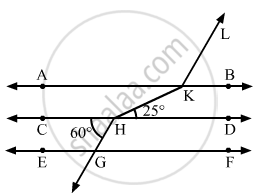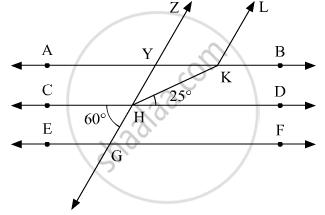Advertisement Remove all ads

# In the Given Figure, Ab || Cd || Ef and Gh || Kl. the Measure of ∠Hkl is - Mathematics

MCQ

In the given figure, AB || CD || EF and GH || KL. The measure of ∠HKL is#### Options

• 85°

• 135°

• 145°

• 215°

Advertisement Remove all ads

#### Solution

The given figure is as follows:Let us extend GH to meet AB at Y.

We have the following:

∠CHG and∠CHY are the supplementary angles. Therefore,

∠CHY +∠CHG =180°

∠CHY = 180° -∠CHG

= 180° - 60°

= 120°

∠CHY = 120°    .... (1)

Since,AB||CD. Thus, ∠DHKand ∠YKHare the interior alternate angles.

Therefore,

∠DHK = ∠YKH

∠YKH = 25°   .... (2)

Since, AB || CD. Thus, ∠CHY and  ∠AYZare the corresponding angles.

Therefore,

∠CHY = ∠AYZ

From (1), we get:

∠AYZ = 120° ……(3)

Since ∠YZ || KL. Thus ∠AYZ and ∠YKL are corresponding angles,

Therefore,

∠AYZ = ∠YKL

∠YKL = 120°     .... (4)

Thus, the required angle x can be calculated as:

x = ∠YKL + ∠YKH

From (3) and (4) we get:

x = 25° + 120°

x= 145°

Concept: Introduction to Lines and Angles
Is there an error in this question or solution?
Advertisement Remove all ads

#### APPEARS IN

RD Sharma Mathematics for Class 9
Chapter 10 Lines and Angles
Q 27 | Page 57
Advertisement Remove all ads
Advertisement Remove all ads
Share
Notifications

View all notifications

Forgot password?
Course